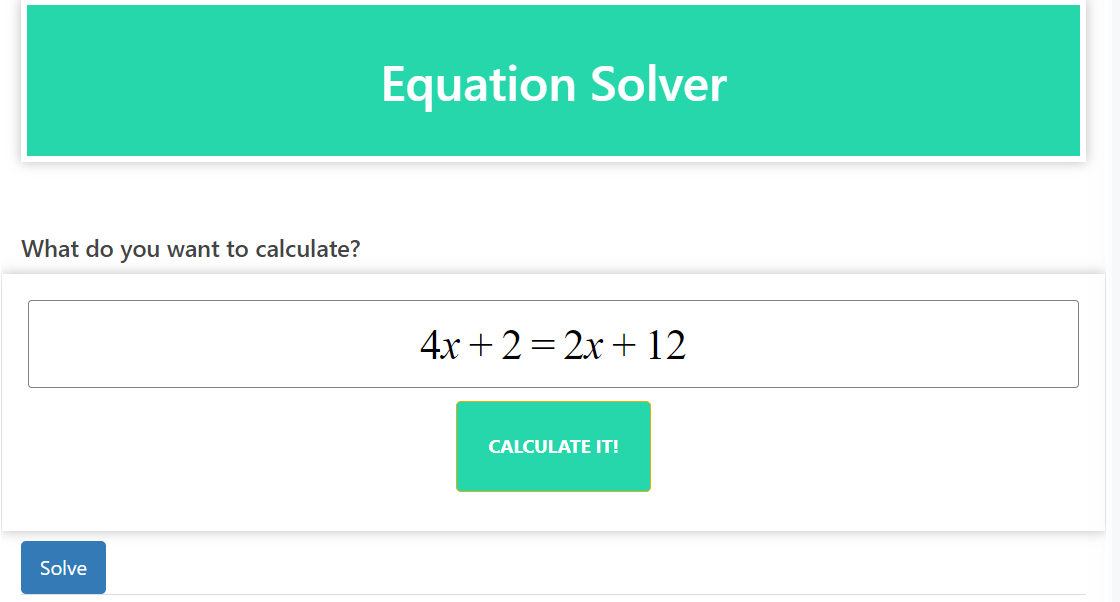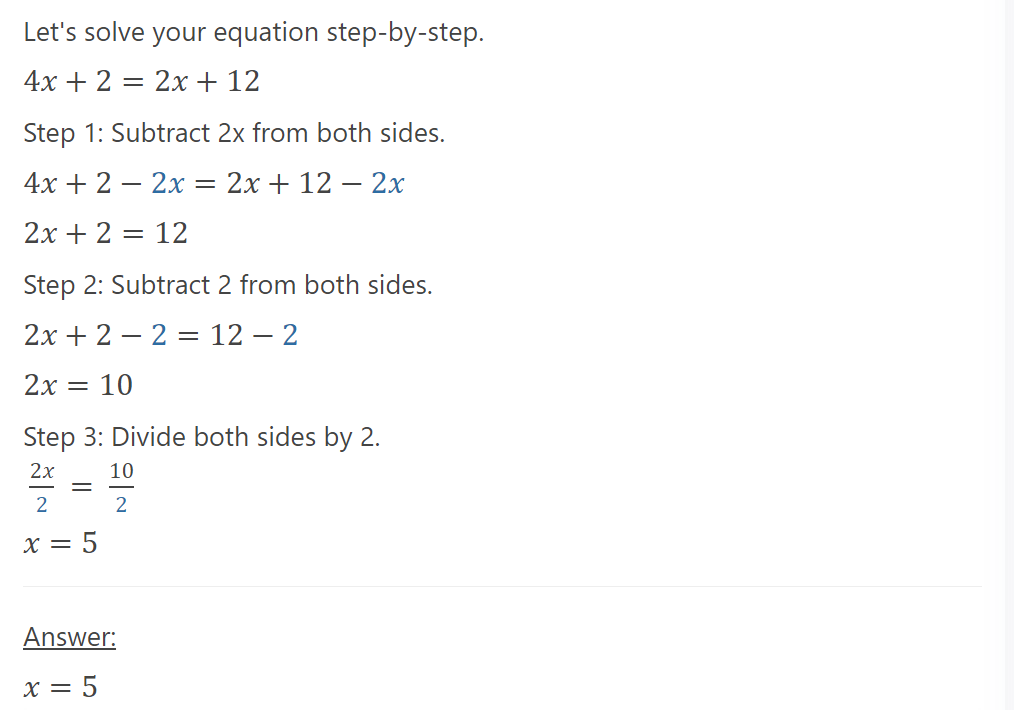# Equation Solver: Unveiling the Power of Solving Complex Equations

Equation Solver is a versatile and indispensable tool that empowers you to conquer the complexities of mathematical equations. Whether you’re faced with linear equations, quadratic equations, or even higher-order polynomials, our Equation Solver is here to provide accurate and efficient solutions.

With our Equations Solver, you can bid farewell to tedious manual calculations and embrace the ease of automated solving. Simply input the equation you want to solve, and let our powerful algorithm work its magic.

The beauty of our Equation Solver lies in its versatility. It can handle a variety of equations across different branches of mathematics, including algebra, calculus, and beyond. No matter the complexity or the number of variables involved, our Equation Solver is up to the task.

### Step-by-Step Solutions:

We understand that the process of solving equations can be just as important as the final result. That’s why our Equations Solver goes beyond providing answers. It walks you through the step-by-step solution, ensuring you gain a deeper understanding of the problem-solving process.

### Efficiency and Accuracy:

Time is of the essence when it comes to solving equations. Our Equations Solver is built to deliver efficient and precise results, allowing you to save valuable time and focus on other critical tasks. Rest assured that our algorithm is designed to provide accurate solutions, giving you the confidence to trust in the results.

### Unlock the Power of Equation Solver:

Whether you’re a student, professional, or enthusiast, our Equations Solver is your reliable companion in tackling mathematical challenges. Harness the power of our intuitive and feature-rich tool to simplify your equation-solving journey.

### Experience the Equations Solver Advantage:

Revolutionize your approach to solving equations with our cutting-edge Equation Solver. Say goodbye to manual labor and hello to efficient, accurate, and step-by-step solutions. Empower yourself with a tool that transforms complex equations into manageable problems. Explore the possibilities with our Equations Solver and unlock your mathematical potential today.